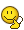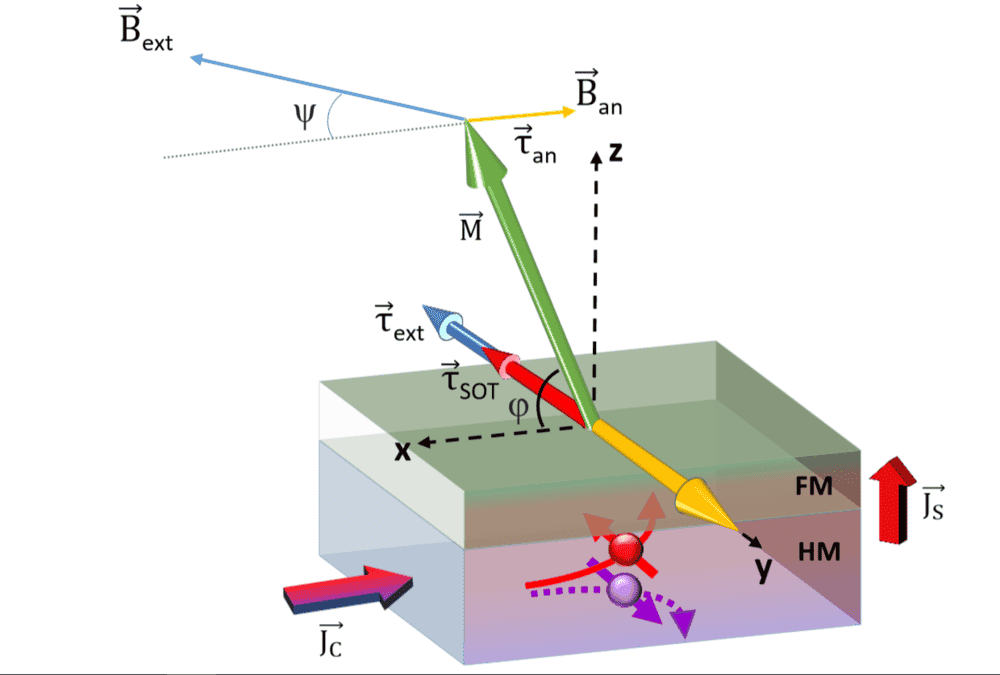# Spin-Orbit-Torque: Where is the damping-like Term in the pic and why?

• JanSpintronics
In summary, the damping torque (\tau_{SOT} in the figure) is perpendicular to the field-like torque (\tau_{ext}), but it is not perpendicular to the original torque (\tau). This might be due to an anisotropy in the material, which is trying to align the magnetization vector in the previous direction.

#### JanSpintronics

Hello everybody,

So my Problem has something to do with Spin Orbit Torques in a Bilayer of a heavy metal and a Ferromagnet. From the Landau-Lifschitz-Gilbert equation we Know that there exists a damping-like and a field-like torque. So far i understand, the damping torque-term is always perpendicular to the one of the fieldlike and always try to damping the field like term, so he have to be perpendicular to the field like Torque. But in the following example its not the case:
Its all decribe in the Macro-Spin-model. In this Bilayer is a Troque coming from the external fiel d, which is given by:
$\tau_{ext}=m \times B_{ext}$
The external field is applied in the xz-plane (not really obviosly in the picture).
The Spin Orbit Troque (SOT) Term, which i know just includes the damping term, is given by:
$\tau_{SOT}= \tau^0 _{SOT}* m\times \sigma \times m$
So and now my questions: As you can see in the picture, the damping term and the Term of the field are pointing in the same direction (the Torques $\tau_{SOT}$ and $\tau_{ext}$). Why is the SOT Term a damping Term?
It looks like he just accelerate or increases the rotation. I would assign the anisotropy-term as a damping term, because this torque is trying to align the magnetization vector in his previous direction (which i understand as a damping effect).
Furthermore I am confused, why this $\tau_{SOT}$ isn't perpendicular to the Torque of the field. i mean, form the LLG-equation the damping term is always a crossproduct from the fieldlike-part-direction and and magnetization vector, so it must be perpendicular to the field-like-term.

I hope you see where i struggle and hopefully you can help me.
Best Regards

PS.sry for my english and sry for posting it in the wrong forum, i didnt find itWelcome to PF!

I had to delete your duplicate post in member intros as we reserve that for intros only.

Alright, sorry again for that, i post a third in a (hopefully right) forumsorry